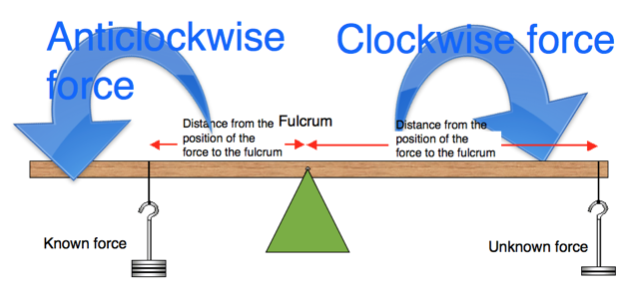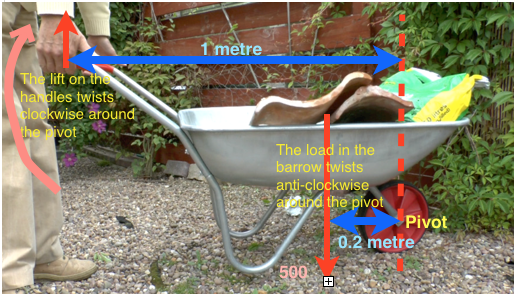### The Law of Moments

This uniform beam is balanced in the centre with a weight on each side.

The Law of Moments states that for equilibrium (balance) the clockwise moments are equal to the anti-clockwise moments.For a video introduction to The Law f Moments, watch the video below.

### Moments Calculations

We can use this idea to find an unknown force.The load in the barrow is 500N and we can calculate the force (F) to just lift (balance) it.

Clockwise moment = F x 1
Anti-clockwise moment = 500 x 0.20

if they are balanced:
F x 1 = 500 x 0.20         so F = 100N

Download PDF of all notes   Moments and the law of moments notes

Other pages on Moments are:

Moments of a Force - explaining what a "moment" is.

Practical examples of where the Law  is used

Calculations